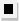Chapter 13.CR, Problem 52E### Algebra and Trigonometry (MindTap ...

4th Edition
James Stewart + 2 others
ISBN: 9781305071742

#### Solutions

Chapter
Section### Algebra and Trigonometry (MindTap ...

4th Edition
James Stewart + 2 others
ISBN: 9781305071742
Textbook Problem

# 4 9 - 5 4Sums of Arithmetic and Geometric Sequences Determine whether the expression is a partial sum of an arithmetic or geometric sequence. Then find the sum. 1 3 + 2 3 + 1 + 4 3 + ⋅ ⋅ ⋅ + 33

To determine

Whether the expression 13+23+1+43++33 is partial sum of arithmetic or geometric sequence. Then find the sum.

Explanation

Given:

The expression is given as,

13+23+1+43++33

Approach:

Check if the terms in the expression have a common difference or a common ratio.

Calculation:

Find the common difference.

2313=13123=13431=13

So there is a common difference of 13.

This proves that the given expression is a partial sum of an arithmetic sequence with first term a=13, common difference, d=13

The nth term in this arithmetic sequence is a+(n1)d=13+(n1)13

We set this equal to 33 to compute number of terms in the expression.

### Still sussing out bartleby?

Check out a sample textbook solution.

See a sample solution

#### The Solution to Your Study Problems

Bartleby provides explanations to thousands of textbook problems written by our experts, many with advanced degrees!

Get Started